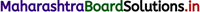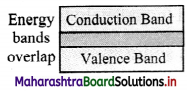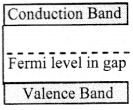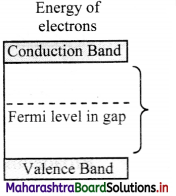# Maharashtra Board Class 11 Physics Solutions Chapter 14 Semiconductors

Balbharti Maharashtra State Board 11th Physics Textbook Solutions Chapter 14 Semiconductors Textbook Exercise Questions and Answers.

## Maharashtra State Board 11th Physics Solutions Chapter 14 Semiconductors

1. Choose the correct option.

Question 1.
Electric conduction through a semiconductor is due to:
(A) Electrons
(B) holes
(C) none of these
(D) both electrons and holes
(D) both electrons and holesQuestion 2.
The energy levels of holes are:
(A) in the valence band
(B) in the conduction band
(C) in the band gap but close to valence band
(D) in the band gap but close to conduction band
(C) in the band gap but close to valence band

Question 3.
Current through a reverse biased p-n junction, increases abruptly at:
(A) Breakdown voltage
(B) 0.0 V
(C) 0.3V
(D) 0.7V
(A) Breakdown voltage

Question 4.
A reverse biased diode, is equivalent to:
(A) an off switch
(B) an on switch
(C) a low resistance
(D) none of the above
(A) an off switch

Question 5.
The potential barrier in p-n diode is due to:
(A) depletion of positive charges near the junction
(B) accumulation of positive charges near the junction
(C) depletion of negative charges near the junction,
(D) accumulation of positive and negative charges near the junction
(D) accumulation of positive and negative charges near the junction

Question 1.
What is the importance of energy gap in a semiconductor?

1. The gap between the bottom of the conduction band and the top of the valence band is called the energy gap or the band gap.
2. This band gap is present only in semiconductors and insulators.
3. Magnitude of the band gap plays a very important role in the electronic properties of a solid.
4. Band gap in semiconductors is of the order of 1 eV.
5. If electrons in valence band of a semiconductor are provided with energy more than band gap energy (in the form of thermal energy or electrical energy), then the electrons get excited and occupy energy levels in conduction band. These electrons can easily take part in conduction.

Question 2.
Which element would you use as an impurity to make germanium an n-type semiconductor?
Germanium can be made an n-type semiconductor by doping it with pentavalent impurity, like phosphorus (P), arsenic (As) or antimony (Sb).Question 3.
What causes a larger current through a p-n junction diode when forward biased?
In case of forward bias the width of the depletion region decreases and the p-n junction offers a low resistance path allowing a high current to flow across the junction.

Question 4.
On which factors does the electrical conductivity of a pure semiconductor depend at a given temperature?
For pure semiconductor, the number density of free electrons and number density of holes is equal. Thus, at a given temperature, the conductivity of pure semiconductor depends on the number density of charge carriers in the semiconductor.

Question 5.
Why is the conductivity of a n-type semiconductor greater than that of p-type semiconductor even when both of these have same level of doping?

1. In a p-type semiconductor, holes are majority charge carriers.
2. When a p-type semiconductor is connected to terminals of a battery, holes, which are not actual charges, behave like a positive charge and get attracted towards the negative terminal of the battery.
3. During transportation of hole, there is an indirect movement of electrons.
4. The drift speed of these electrons is less than that in the n-type semiconductors. Mobility of the holes is also less than that of the electrons.
5. As, electrical conductivity depends on the mobility of charge carriers, the conductivity of a n-type semiconductor is greater than that of p-type semiconductor even when both of these have same level of doping.

Question 1.
Explain how solids are classified on the basis of band theory of solids.
i. The solids can be classified into conductors, insulators and semiconductors depending on the distribution of electron energies in each atom.

ii. As an outcome of the small distances between atoms, the resulting interaction amongst electrons and the Pauli’s exclusion principle, energy bands are formed in the solids.

iii. In metals, conduction band and valence band overlap. However, in a semiconductor or an insulator, there is gap between the bottom of the conduction band and the top of the valence band. This is called the energy gap or the band gap.iv. For metals, the valence band and the conduction band overlap and there is no band gap as shown in figure (b). Therefore, electrons can easily gain electrical energy when an external electric field is applied and are easily available for conduction.v. In case of semiconductors, the band gap is fairly small, of the order of 1 eV or less as shown in figure (c). Hence, with application of external electric field, electrons get excited and occupy energy levels in conduction band. These can take part in conduction easily.vi. Insulators, on the contrary, have a wide gap between valence band and conduction band of the order of 5 eV (for diamond) as shown in figure (d). Therefore, electrons find it very difficult to gain sufficient energy to occupy energy levels in conduction band.vii. Thus, an energy band gap plays an important role in classifying solids into conductors, insulators and semiconductors based on band theory of solids.Question 2.
Distinguish between intrinsic semiconductors and extrinsic semiconductors

 Intrinsic semiconductors Extrinsic semiconductors 1. A pure semiconductor is known as intrinsic semiconductors. The semiconductor, resulting 2. Their conductivity is low Their conductivity is high even at room temperature. 3. Its electrical conductivity is a function of temperature alone. Its electrical conductivity depends upon the temperature as well as on the quantity of impurity atoms doped in the structure. 4. The number density of holes (nh) is same as the number density of free electron (ne) (nh = ne). The number density of free electrons and number density of holes are unequal.

Question 3.
Explain the importance of the depletion region in a p-n junction diode.
i. The region across the p-n junction where there are no charges is called the depletion layer or the depletion region.

ii. During diffusion of charge carriers across the junction, electrons migrate from the n-side to the p-side of the junction. At the same time, holes are transported from p-side to n-side of the junction.

iii. As a result, in the p-type region near the junction there are negatively charged acceptor ions, and in the n-type region near the junction there are positively charged donor ions.

iv. The potential barrier thus developed, prevents continuous flow of charges across the junction. A state of electrostatic equilibrium is thus reached across the junction.

v. Free charge carriers cannot be present in a region where there is a potential barrier. This creates the depletion region.

vi. In absence of depletion region, all the majority charge carriers from n-region (i.e., electron) will get transferred to the p-region and will get combined with the holes present in that region. This will result in the decreased efficiency of p-n junction.

vii. Hence, formation of depletion layer across the junction is important to limit the number of majority carriers crossing the junction.

Question 4.
Explain the I-V characteristic of a forward biased junction diode.1. Figure given below shows the I-V characteristic of a forward biased diode.
2. When connected in forward bias mode, initially, the current through diode is very low and then there is a sudden rise in the current.
3. The point at which current rises sharply is shown as the ‘knee’ point on the I-V characteristic curve.
4. The corresponding voltage is called the knee voltage. It is about 0.7 V for silicon and 0.3 V for germanium.
5. A diode effectively becomes a short circuit above this knee point and can conduct a very large current.
6. To limit current flowing through the diode, resistors are used in series with the diode.
7. If the current through a diode exceeds the specified value, the diode can heat up due to the Joule’s heating and this may result in its physical damage.Question 5.
Discuss the effect of external voltage on the width of depletion region of a p-n junction.

1. A p-n junction can be connected to an external voltage supply in two possible ways.
2. A p-n junction is said to be connected in a forward bias when the p-region connected to the positive terminal and the n-region is connected to the negative terminal of an external voltage source.
3. In forward bias connection, the external voltage effectively opposes the built-in potential of the junction. The width of depletion region is thus reduced.
4. The second possibility of connecting p-n junction is in reverse biased electric circuit.
5. In reverse bias connection, the p-region is connected to the negative terminal and the n-region is connected to the positive terminal of the external voltage source. This external voltage effectively adds to the built-in potential of the junction. The width of potential barrier is thus increased

11th Physics Digest Chapter 14 Semiconductors Intext Questions and Answers

Internet my friend (Textbookpage no. 256)

i. https://www.electronics-tutorials.ws/diode
ii. https://www.hitachi-hightech.com
iii. https://nptel.ac.in/courses
iv. https://physics.info/semiconductors
v. http://hyperphysics.phy- astr.gsu.edu/hbase/Solids/semcn.html